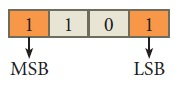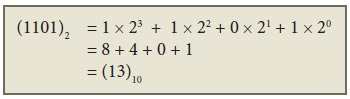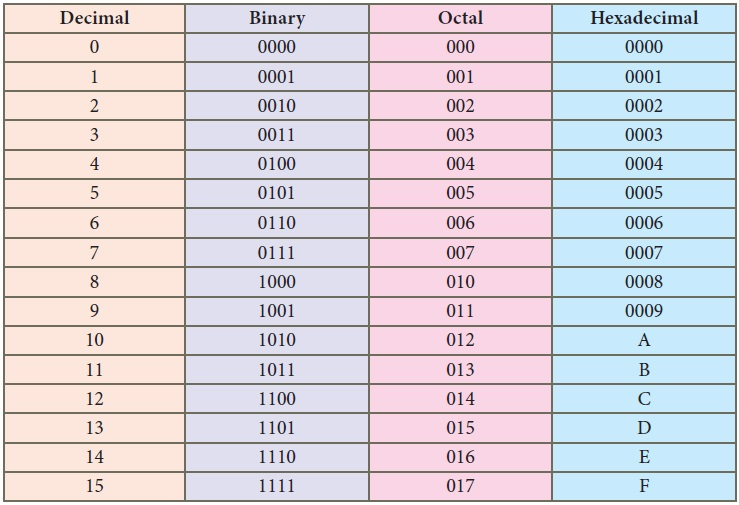Home | | Computer Technology 11th std | Different Types of Number Systems

# Different Types of Number Systems

A numbering system is a way of representing numbers.

Different Types of Number SystemsA numbering system is a way of representing numbers. The most commonly used numbering system in real life is Decimal number system. Other number systems are Binary, Octal, Hexadecimal number system. Each number system is uniquely identified by its base value or radix. Radix or base is the count of number of digits in each number system. Radix or base is the general idea behind positional numbering system.

## 1. Decimal Number System

It consists of 0,1,2,3,4,5,6,7,8,9(10 digits). It is the oldest and most popular number system used in our day to day life. In the positional number system, each decimal digit is weighted relative to its position in the number. This means that each digit in the number is multiplied by 10 raised to a power corresponding to that digit’s position.

### Example## 2. Binary Number System

There are only two digits in the Binary system, namely, 0 and 1. The numbers in the binary system are represented to the base 2 and the positional multipliers are the powers of 2. The left most bit in the binary number is called as the Most Significant Bit (MSB) and it has the largest positional weight. The right most bit is the Least Significant Bit (LSB) and has the smallest positional weight.### Example

The binary sequence (1101)2 has the decimal equivalent:## 3. Octal Number System

Octal number system uses digits 0,1,2,3,4,5,6 and 7 (8 digits). Each octal digit has its own positional value or weight as a power of 8.

### Example

The Octal sequence (547)8 has the decimal equivalent:A hexadecimal number is represented using base 16. Hexadecimal or Hex numbers are used as a shorthand form of binary sequence. This system is used to represent data in a more compact manner. Since 16 symbols are used, 0 to F, the notation is called hexadecimal. The first 10 symbols are the same as in the decimal system, 0 to 9 and the remaining 6 symbols are taken from the first 6 letters of the alphabet sequence, A to F, where A represents 10, B is 11, C is 12, D is 13, E is 14 and F is 15.

Table 2.2 Binary, Octal, Hexadecimal equivalent of Decimal Numbers### Example

The hexadecimal sequence (25)16 has the decimal equivalent:Study Material, Lecturing Notes, Assignment, Reference, Wiki description explanation, brief detail
11th Computer Science : Chapter 2 : Number Systems : Different Types of Number Systems |